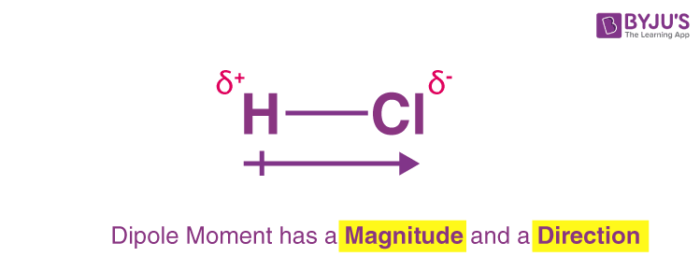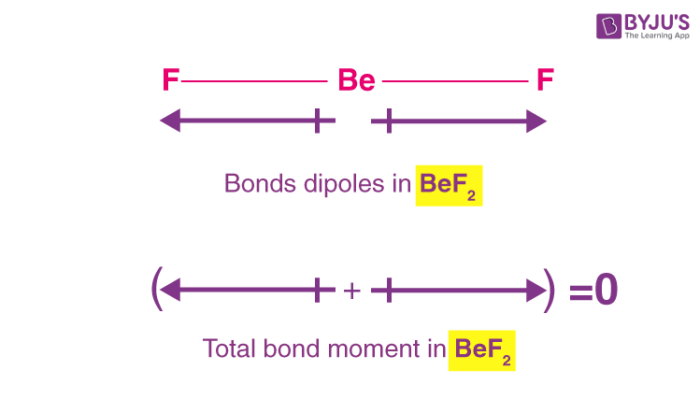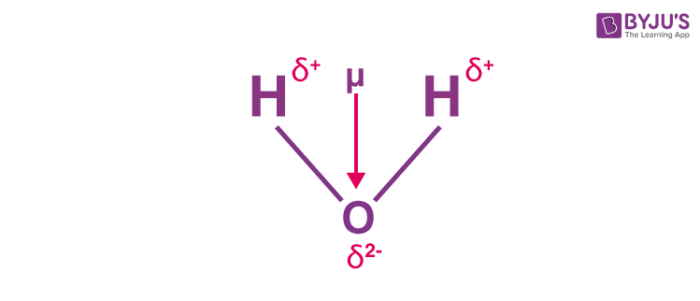# Dipole Moment

## What is Dipole Moment?

A dipole moment arises in any system in which there is a separation of charge. They can, therefore, arise in ionic bonds as well as in covalent bonds. Dipole moments occur due to the difference in electronegativity between two chemically bonded atoms.

A bond dipole moment is a measure of the polarity of a chemical bond between two atoms in a molecule. It involves the concept of electric dipole moment, which is a measure of the separation of negative and positive charges in a system.

The bond dipole moment is a vector quantity since it has both magnitude and direction. An illustration describing the dipole moment that arises in an HCl (hydrochloric acid) molecule is provided below.It can be noted that the symbols 𝛿+ and 𝛿 represent the two electric charges that arise in a molecule which are equal in magnitude but are of opposite signs. They are separated by a set distance, which is commonly denoted by ‘d’.

### Important Points

• The dipole moment of a single bond in a polyatomic molecule is known as the bond dipole moment and it is different from the dipole moment of the molecule as a whole.
• It is a vector quantity, i.e. it has magnitude as well as definite directions.
• Being a vector quantity, it can also be zero as the two oppositely acting bond dipoles can cancel each other.
• By convention, it is denoted by a small arrow with its tail on the negative center and its head on the positive center.
• In chemistry, the dipole moment is represented by a slight variation of the arrow symbol. It is denoted by a cross on the positive center and arrowhead on the negative center. This arrow symbolizes the shift of electron density in the molecule.
• In the case of a polyatomic molecule, the dipole moment of the molecule is the vector sum of the all present bond dipoles in the molecule.

## Recommended Videos## Dipole Moment Formula

A dipole moment is the product of the magnitude of the charge and the distance between the centers of the positive and negative charges. It is denoted by the Greek letter ‘µ’.

Mathematically,

Dipole Moment (µ) = Charge (Q) * distance of separation (r)

It is measured in Debye units denoted by ‘D’. 1 D = 3.33564 × 10-30 C.m, where C is Coulomb and m denotes a metre.

The bond dipole moment that arises in a chemical bond between two atoms of different electronegativities can be expressed as follows:

μ = 𝛿.d

Where: μ is the bond dipole moment,

𝛿 is the magnitude of the partial charges 𝛿+ and 𝛿,

And d is the distance between 𝛿+ and 𝛿.

The bond dipole moment (μ) is also a vector quantity, whose direction is parallel to the bond axis. In chemistry, the arrows that are drawn in order to represent dipole moments begin at the positive charge and end at the negative charge.

When two atoms of varying electronegativities interact, the electrons tend to move from their initial positions to come closer to the more electronegative atom. This movement of electrons can be represented via the bond dipole moment.

## Examples

### Dipole moment of BeF2

In a beryllium fluoride molecule, the bond angle between the two beryllium-fluorine bonds is 180o. Fluorine, being the more electronegative atom, shifts the electron density towards itself. The individual bond dipole moments in a BeF2 molecule are illustrated below.From the illustration provided above, it can be understood that the two individual bond dipole moments cancel each other out in a BeF2 molecule because they are equal in magnitude but are opposite in direction. Therefore, the net dipole moment of a BeF2 molecule is zero.

### Dipole moment of H2O (Water)

In a water molecule, the electrons are localised around the oxygen atom since it is much more electronegative than the hydrogen atom. However, the presence of a lone pair of electrons in the oxygen atom causes the water molecule to have a bent shape (as per the VSEPR theory). Therefore, the individual bond dipole moments do not cancel each other out as is the case in the BeF2 molecule. An illustration describing the dipole moment in a water molecule is provided below.The bond angle in a water molecule is 104.5o. The individual bond moment of an oxygen-hydrogen bond is 1.5 D. The net dipole moment in a water molecule is found to be 1.84D.

## Frequently Asked Questions – FAQs

Q1

### Why is it called a dipole moment?

A dipole moment is a measurement of the separation of two opposite electrical charges. Dipole moments are a vector quantity. The magnitude is equal to the charge multiplied by the distance between the charges and the direction is from negative charge to positive charge:
μ = q · r
where μ is the dipole moment, q is the magnitude of the separated charge, and r is the distance between the charges.’

Q2

### How do you find the largest dipole moment?

When there is a difference in the electronegativity of two atoms involved in a bond, a dipole moment happens. The larger the electronegativity difference between the two atoms, the larger the bond’s dipole moment and polarity.

Q3

### How do you find the dipole moment of co2?

Carbon dioxide has a linear geometry at the core with carbon and oxygen on both sides while oxygen is more electronegative than carbon electron cloud is pushed to oxygen and both oxygen atom the electron cloud from both sides with the same tendency, so the net effect is zero.

Q4

### What is the symbol of dipole moment?

The dipole moment (μ) is the calculation of the net molecular polarity at either end of the molecular dipole, which is the magnitude of the charge Q times the distance r between the charges. Dipole moment tells about charge separation in a molecule.

Test your Knowledge on Dipole moment!

1. nicely explained . thanks

2. Awesome

3. Thanks quite simple, comprehensive and interesting

4. thanks for this valuable information

5. it is quite simply explained. thank u for this

6. good explaination. Thank u

7. clear and comprehensive explanatory